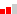(0 Rating)Free
Message Instructor

Highlights

• Over 5 hours of instruction
• Excellent Instructors
• Convenient: Learn Anywhere!
Certificate

No

Level

IntermediateLanguage

English (US)

Contents:

- Understanding Tenths, Hundredths, and Thousandths

- Fractions and Decimals (Expressing Decimals as Fractions and Fractions as Decimals)

- Comparing an Ordering Decimals

- Number patterns

- Rounding Decimals

- Subtracting Decimals

- Multiplying Decimals by a 1-digit whole number

- Dividing Decimals by a 1-digit whole number

- Word Problems

Who can join:

- If you are using the Singapore Mathematics Curriculum and would like enrichment and supplemental material.

- If you would like to learn math using the Singapore Mathematics strategies.

Singapore Mathematics Curriculum:

Math in Focus: Chapter 7/8

Math in Focus 2020: Chapter 4

Primary Mathematics US Ed.: Chapter 8/9

Dimensions Math: Chapter 12/13/1

• Private